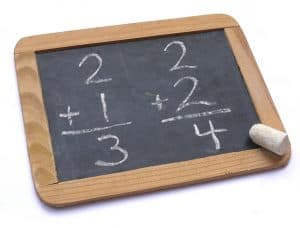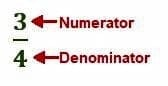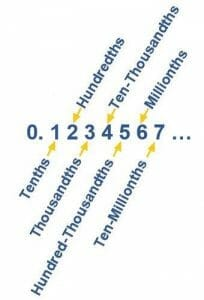# ArithmeticThe “Three R’s” of education – Reading, Writing, and ‘Rithmetic – have long been considered the basis for almost all schooling.

Arithmetic, in particular, provides the foundation for most of mathematics, including algebra, geometry, statistics, and calculus.

In general, arithmetic deals with all of the basic notions of numbers, including place value and comparisons. It also includes the fundamental operations of addition, subtraction, multiplication, division and their properties. Relationships between fractions and decimals are also considered part of arithmetic.

This guide provides resources for reviewing and learning arithmetic topics ranging from elementary arithmetic, namely whole numbers and fundamental operations, to more complex ones such as negative numbers and proportions. Since the topics build on each other, it is best to move through them in order beginning with whole numbers and ending with word problems.

Each topic here will contain a brief explanation followed by links to resources on subtopics. These subtopics also build on each other, so again it is best to move through them in order.

## Whole Numbers

Whole numbers are technically defined as numbers that do not have a fractional part. Most people just think of them as the “counting numbers,” 1, 2, 3, etc. Knowing the properties of whole numbers is integral to understanding the relationships between numbers more generally.

The following subtopics begin with the place value of whole numbers and move through the fundamental arithmetic operations and their properties.

## Numbers

Numbers represent values. The notion includes both real and complex numbers, rational and irrational numbers, positive and negative numbers, whole numbers, and zero.

The subtopics here begin with an explanation and comparison of different types of numbers and then move to properties of numbers.

These properties are then used to explain other qualities of numbers such as greatest common factors. Finally, this section ends with an explanation of roots and how to work with radicals.

## Positive and Negative Integers

Integers include all positive whole numbers, their negative counterparts, and zero. That is, they include all numbers that do not have a fractional part.

## Fractions

Fractions are a representation of a ratio between two numbers. They are written in terms of one number divided by another number. The top number is called a numerator, and the non-zero bottom number is called a denominator.

The numerator and denominator are separated by a small line called a fraction bar, which represents division. Any number that can be written as a fraction is called a rational number.This topic begins by introducing fractions, their relative values, and different ways to write them. It then moves on to operations with fractions and mixed numbers. It ends with a brief introduction of how to convert fractions into decimals, which will be further explained in the following topic about decimals.

## DecimalsDecimals represent parts of a whole number using powers of ten. A decimal point is placed to the right of the ones place in any number, and any digit to the right of that is considered a “decimal part” of that number.

This topic begins with a guide to understanding and using decimal place value. It then moves on to understanding decimal operations and converting decimals to fractions.

## Exponents

Just as multiplication is an operation that defines repeated addition, exponents define repeated multiplication.

This operation is signified by placing one number, called the exponent, to the upper right of another number, called the base. The exponent shows how many times the base is to be multiplied by itself. This is read as “[the base] raised to the power of [the exponent].”After explaining how exponents work, this topic goes over different types of exponents, such as those with fractions and negative numbers. It ends with guides to exponent operations.

## Percent

The English word “percent” comes from the Latin “per centum” meaning “by one hundred.” A percent is another way of representing fractional part of a number. In particular, it is the representation of something out of one hundred.Since percents are a representation of a part of a whole, this subtopic begins with the relationship between percents and fractions and decimals. It then covers some of the ways in which percentages are used in real life, including the simple interest formula.

## Scientific Notation

Scientific notation is a way to efficiently write very large or very small numbers using powers of 10. Unsurprisingly, it is often used in science where its ability to denote the precision of a measurement and easily compare numbers gives it an advantage over standard form.

This topic first explains scientific notation and how to convert back and forth to standard form. It then explains how to do basic operations on numbers in scientific notation without first converting to standard form.

## Proportion and Variation

Proportion and variation are ways of describing the relationship between two quantities using ratios.

This topic goes over ratios, proportions, and variations. It then explains the differences between relationships that are directly and inversely proportional.